Home > CC4 > Chapter A > Lesson A.1.5 > ProblemA-54

A-54.

A-54. Simplify each expression. Homework Help ✎

1.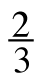+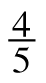2. 2 −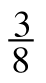3.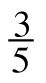· 10

4.÷ −2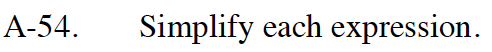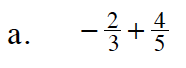Multiply by fractions equal to one to make the denominators the same.

$-\frac{2}{3}\cdot \frac{5}{5}+\frac{4}{5}\cdot \frac{3}{3}$

$=\ -\frac{10}{15}+ \frac{12}{15}$

$\frac{2}{15}$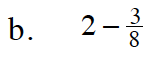Change 2 into a fraction greater than one.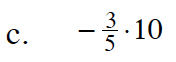Simplify before multiplying.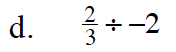Multiply by the reciprocal.

$-\frac{1}{3}$